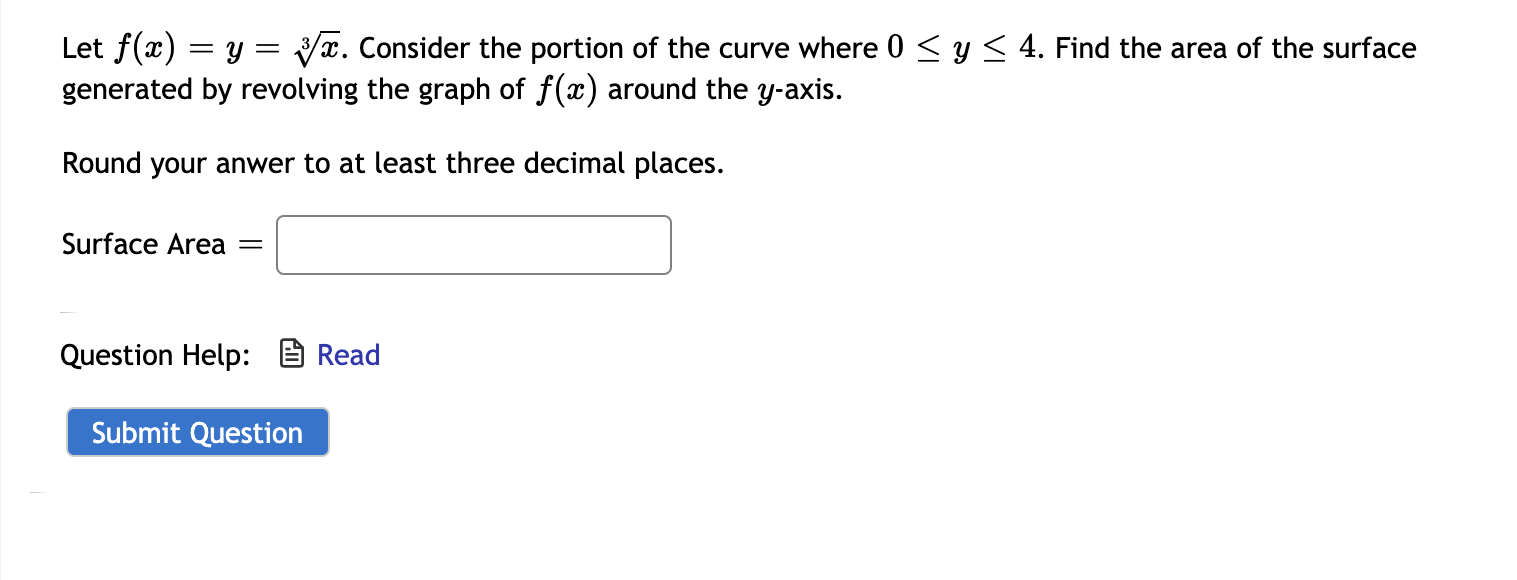# Let f(x) = y = yx. Consider the portion of the curve where 0 < y< 4. Find the area of the surface generated by revolving the graph of f(x) around the y-axis. Round your anwer to at least three decimal places. Surface Area Question Help: B Read

Questionhelp_outlineImage TranscriptioncloseLet f(x) = y = yx. Consider the portion of the curve where 0 < y< 4. Find the area of the surface generated by revolving the graph of f(x) around the y-axis. Round your anwer to at least three decimal places. Surface Area Question Help: B Read fullscreen

### Want to see this answer and more?

Experts are waiting 24/7 to provide step-by-step solutions in as fast as 30 minutes!*

*Response times may vary by subject and question complexity. Median response time is 34 minutes for paid subscribers and may be longer for promotional offers.
Tagged in
MathCalculus

### Applications of Integration